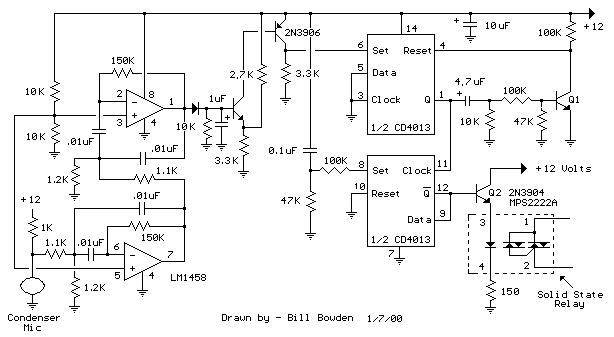# 口哨声控制开关 声控开关

R1 = Q /（G * C * 2 * PI * F）= 8 /（65 * 0.01 ^ -6 * 6.28 * 1700）= 1152或1.1K
R2 = Q /（（2 * Q ^ 2）-G）* C * 2 * PI * F）= 8 /（（128-65）* 0.01 ^ -6 * 6.28 * 1700）= 1189或1.2K
R3 =（2 * Q）/（C * 2 * PI * F）= 16 /（0.01 ^ 6 * 6.28 * 1700）= 150K